12 Rates

# Multiple Volume Expansion method

Selective CO2 measurement can be done with the Multiple Volume Expansion method (MVE method).The MVE method was invented and patented by Anton Paar (AT 409673, GB 237 3584, US 6,874,351). It is the most accurate way of selectively measuring dissolved CO2 in beverages.

## Fundamental gas laws relevant for CO₂ measurement in beverages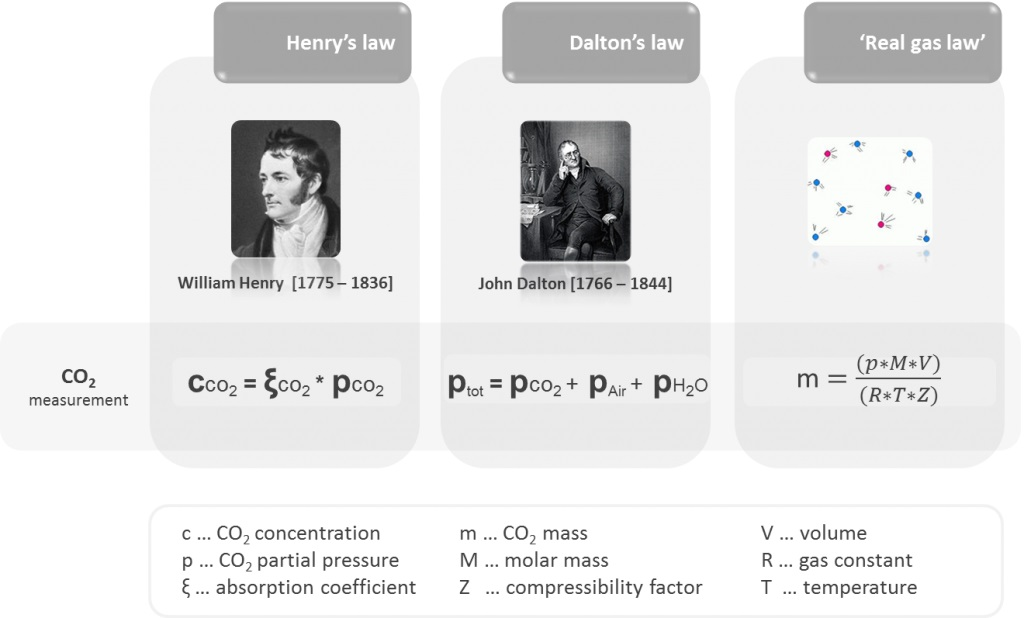Figure 1: Overview of the fundamental gas laws which are relevant for CO2 measurement

Henry‘s law states that at equilibrium the concentration of dissolved gas in a liquid is proportional to the partial pressure of that gas above the liquid.

Dalton‘s law states that the (total) pressure measured in a gas phase is the sum of the partial pressures of all gases present in the gas phase. In the case of beverage analysis, these are the carbon dioxide, oxygen, and nitrogen partial pressures as well the vapor pressure of water.

The real gas law describes the behavior of an ideal gas in the gas phase. As gases like CO2 deviate from the ideal behavior, the gas law is expanded by Z to consider the non-ideal behavior.

## How does the Multiple Volume Expansion method work?

#### 1st Step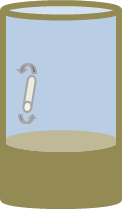The measuring chamber is completely filled with sample and mechanically closed by valves.

#### 2nd Step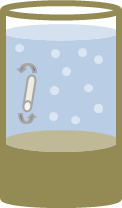The volume of the measuring chamber is expanded. Pressure and temperature equilibrium is generated. Equilibrium pressure and temperature are measured.

#### 3rd Step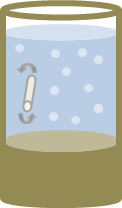The measuring chamber volume is further expanded, equilibrium is generated, and pressure and temperature are measured again.

#### Final Step

The two pressures/temperatures are used for CO2 determination and dissolved air compensation.

## Why does the Multiple Volume Expansion method work?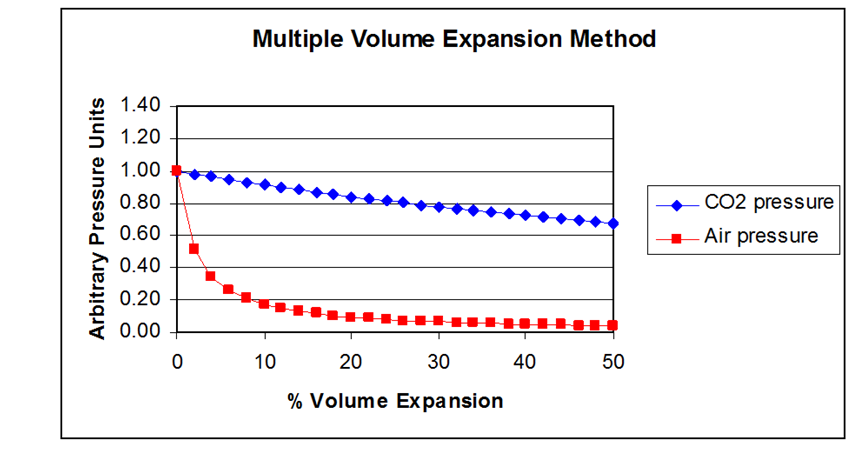Figure 2: Physical background of the MVE method

The patented Multiple Volume Expansion method makes use of the fact that the solubility of air in beverages is much lower than that of CO2.

Due to the difference in solubility, when expanding the volume of the measuring chamber, the partial pressure of air decreases much more than that of CO2.

The difference between the equilibrium pressure and temperature results measured at the first and second volume expansions of the measuring chamber is used to determine the amount of dissolved air and mathematically calculate and compensate for this amount. The result is the true CO2 concentration in the beverage.

## Benefits

### The MVE method is not influenced by snifting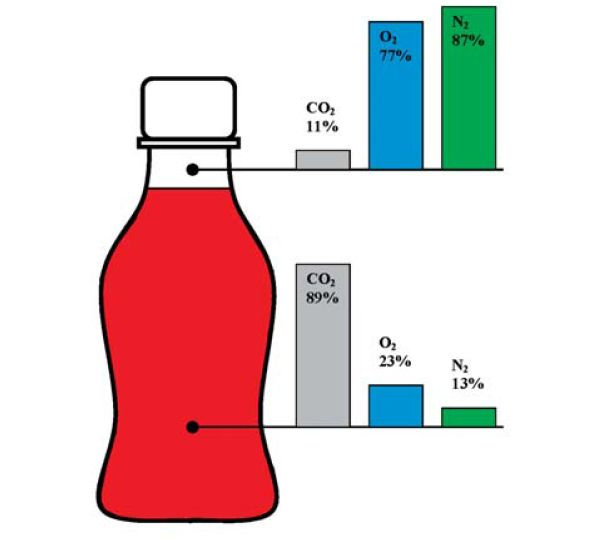Figure 3: Distribution of gases in a package with 10 % headspace at approx. 20 °C (68 °F)

Traditional pressure/temperature (P/T) methods require snifting. This is done by releasing the overpressure in the headspace to the ambience in order to correct the influence of other gases; BUT CO2 also gets released.Both the CO2 and the air loss are temperature-dependent.

Both the CO2 and the air loss are affected by the size of the headspace and the snifting time. Thus, variable amounts of CO2, O2, and N2 are removed from the package prior to the equilibration and P/T measurement.

### MVE method is not influenced by methods and tables

Traditional P/T methods require tables for the CO2 calculation.

This fact leads to inaccurate results on the one hand as interpolating is sometimes necessary (e.g. pressure reading of 21 psi, but only 20 and 22 psi are available in the table), and on the other hand it makes the measurement itself user-dependent due to many manual steps.

### The MVE method is not influenced by altitude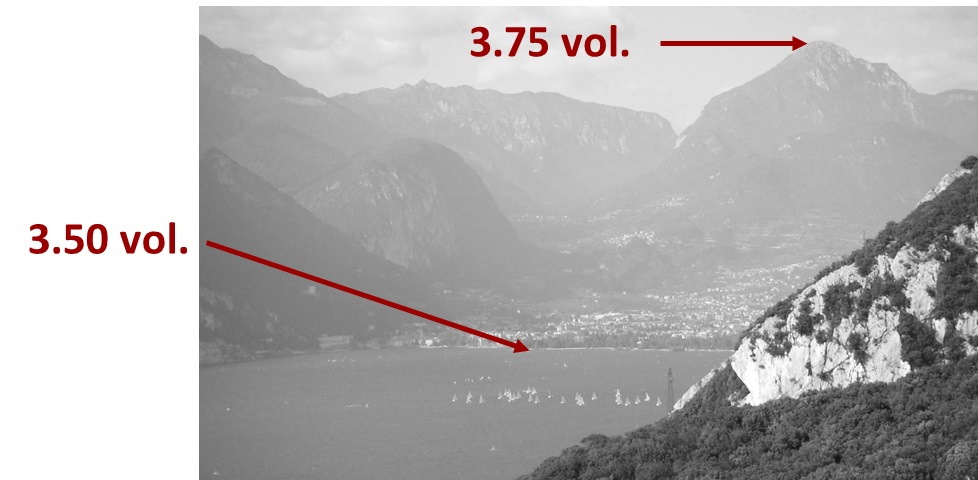Figure 4: Schematic representation of the influence of altitude on single P/T methods

The ambient air pressure at an altitude of 1000 m is about 10 % smaller than at sea level.
When snifting a beverage package at a high altitude, more CO2 and air will be lost to the ambience.
Therefore, the CO2 value of nonselective P/T methods is influenced by altitude.

This gets even worse if a relative pressure sensor is used because then also the pressure value measured in the beverage headspace becomes altitude-dependent.

### The MVE method is not influenced by dissolved air (N₂ and O₂) in the sample

In nonselective single P/T methods, the partial pressures of both CO2 and air are calculated as CO2.
The partial pressure of air depends on the filling technique: Filling with N2 cover gas will increase the CO2 result, filling with CO2 cover gas will not affect the result.

Conclusion: If there is no CO2 in the package, but N2, the pressure gauge will still give a reading even though there is no CO2 in the beverage container.